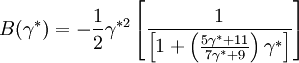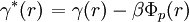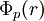# Duh Haymet

Jump to: navigation, search

The Duh-Haymet (Ref. 1) (1995) Padé (3/2) approximation for the bridge function for the Lennard-Jones system is (Eq. 13)$B(\gamma^{*})= - \frac{1}{2} \gamma^{*2} \left[ \frac{1}{ \left[ 1+ \left( \frac{5\gamma^{*} +11}{7\gamma^{*} +9} \right) \gamma^{*} \right]} \right]$

where (Eq. 10)$\left. \gamma^{*}(r) \right.= \gamma (r) - \beta \Phi_p(r)$

where$\Phi_p (r)$ is the perturbative (attractive) part of the pair potential.